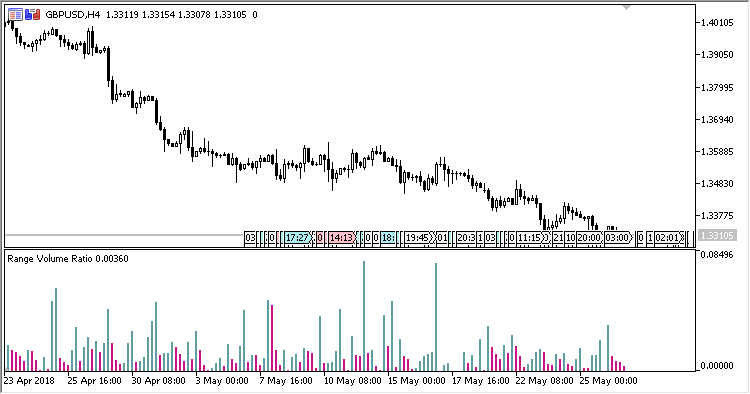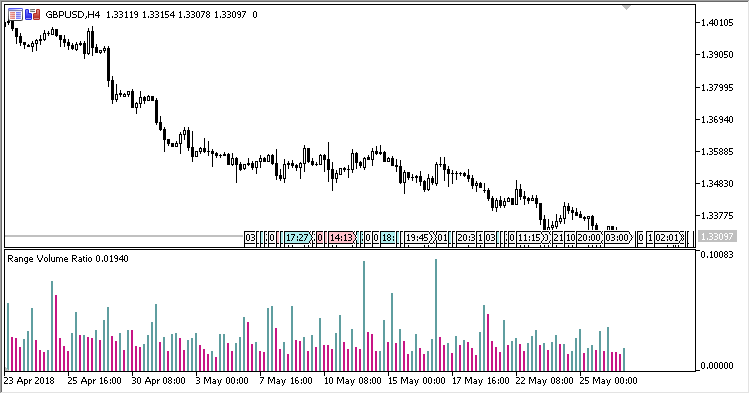Interesting script?
So post a link to it -
let others appraise it

You liked the script? Try it in the MetaTrader 5 terminal# Range_Volume_Ratio - indicator for MetaTrader 5

Views:
1082
Rating:
Published:
2018.06.18 16:23

The indicator shows in a separate window, as a histogram, the candlestick size / tick volume ratios:

1. Difference between Open and Close;
2. Difference between High and Low.

The indicator has one input parameter:

• Calculation type - type of calculations:
• Open/Close - difference between Open and Close;
• High/Low - difference between High and Low.

Calculations:

If the calculation type is Open/Close:

`RVR[i] = Abs(Open[i] - Close[i]) / Volume[i]`

If the calculation type is High/Low:

`RVR[i] = (High[i] - Low[i]) / Volume[i]`

where:

`Volume - the tick volume of the candlestick`Fig.1. Calculations by Open/CloseFig.1. Calculations by High/Low

Translated from Russian by MetaQuotes Software Corp.
Original code: https://www.mql5.com/ru/code/20921Range_Histogram

A candlestick size indicator.Rainbow_Volume

A colored tick-volume indicator.Range_Volume_Ratio_S

Indicator of candlestick size / tick volume ratios and signal line.RVRResistance

An indicator of the volume / bar price range ratio with a signal line and with the option of identifying the maximum/minimum price change resistance.